# 现代 C++：一文读懂智能指针

2021-09-25 184点热度 0人点赞 0条评论

## 智能指针

C++11 引入了 3 个智能指针类型：

1. std::unique_ptr ：独占资源所有权的指针。
2. std::shared_ptr ：共享资源所有权的指针。
3. std::weak_ptr ：共享资源的观察者，需要和 std::shared_ptr 一起使用，不影响资源的生命周期。

std::auto_ptr 已被废弃。

## std::unique_ptr

std::unique_ptr 的使用比较简单，也是用得比较多的智能指针。这里直接看例子。

1. 使用裸指针时，要记得释放内存。
{
int* p = new int(100);
// ...
delete p;  // 要记得释放内存
}

1. 使用 std::unique_ptr 自动管理内存。
{
std::unique_ptr<int> uptr = std::make_unique<int>(200);
//...
// 离开 uptr 的作用域的时候自动释放内存
}

1. std::unique_ptr 是 move-only 的。
{
std::unique_ptr<int> uptr = std::make_unique<int>(200);
std::unique_ptr<int> uptr1 = uptr;  // 编译错误，std::unique_ptr<T> 是 move-only 的

std::unique_ptr<int> uptr2 = std::move(uptr);
assert(uptr == nullptr);
}

1. std::unique_ptr 可以指向一个数组。
{
std::unique_ptr<int[]> uptr = std::make_unique<int[]>(10);
for (int i = 0; i < 10; i++) {
uptr[i] = i * i;
}
for (int i = 0; i < 10; i++) {
std::cout << uptr[i] << std::endl;
}
}

1. 自定义 deleter。
{
struct FileCloser {
void operator()(FILE* fp) const {
if (fp != nullptr) {
fclose(fp);
}
}
};
std::unique_ptr<FILE, FileCloser> uptr(fopen("test_file.txt", "w"));
}

1. 使用 Lambda 的 deleter。
{
std::unique_ptr<FILE, std::function<void(FILE*)>> uptr(
fopen("test_file.txt", "w"), [](FILE* fp) {
fclose(fp);
});
}


## std::shared_ptr

std::shared_ptr 其实就是对资源做引用计数——当引用计数为 0 的时候，自动释放资源。

{
std::shared_ptr<int> sptr = std::make_shared<int>(200);
assert(sptr.use_count() == 1);  // 此时引用计数为 1
{
std::shared_ptr<int> sptr1 = sptr;
assert(sptr.get() == sptr1.get());
assert(sptr.use_count() == 2);   // sptr 和 sptr1 共享资源，引用计数为 2
}
assert(sptr.use_count() == 1);   // sptr1 已经释放
}
// use_count 为 0 时自动释放内存


{
// C++20 才支持 std::make_shared<int[]>
// std::shared_ptr<int[]> sptr = std::make_shared<int[]>(100);
std::shared_ptr<int[]> sptr(new int);
for (int i = 0; i < 10; i++) {
sptr[i] = i * i;
}
for (int i = 0; i < 10; i++) {
std::cout << sptr[i] << std::endl;
}
}

{
std::shared_ptr<FILE> sptr(
fopen("test_file.txt", "w"), [](FILE* fp) {
std::cout << "close " << fp << std::endl;
fclose(fp);
});
}


## std::shared_ptr 的实现原理

  std::cout << sizeof(int*) << std::endl;  // 输出 8  std::cout << sizeof(std::unique_ptr<int>) << std::endl;  // 输出 8  std::cout << sizeof(std::unique_ptr<FILE, std::function<void(FILE*)>>)            << std::endl;  // 输出 40  std::cout << sizeof(std::shared_ptr<int>) << std::endl;  // 输出 16  std::shared_ptr<FILE> sptr(fopen("test_file.txt", "w"), [](FILE* fp) {    std::cout << "close " << fp << std::endl;    fclose(fp);  });   std::cout << sizeof(sptr) << std::endl;  // 输出 16


shared_ptr 需要维护的信息有两部分：

1. 指向共享资源的指针。
2. 引用计数等共享资源的控制信息——实现上是维护一个指向控制信息的指针。

std::shared_ptr<T> sptr1(new T);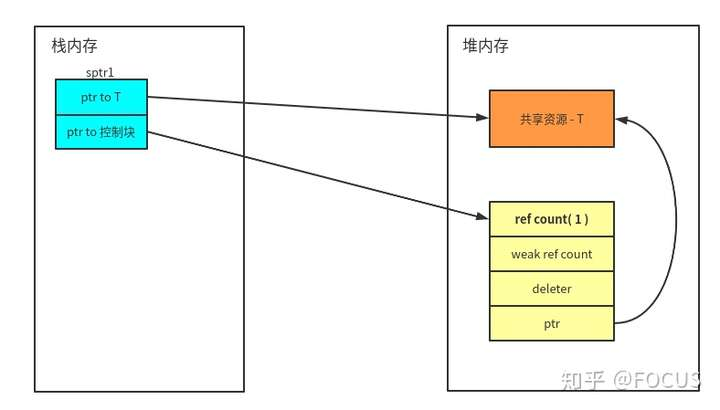std::shared_ptr<T> sptr2 = sptr1;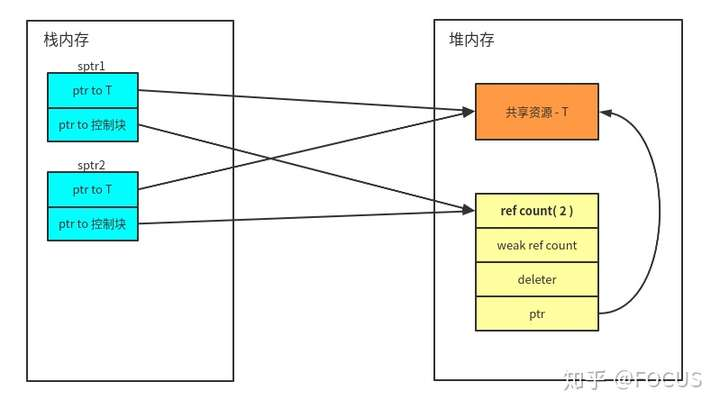struct Fruit {    int juice;};struct Vegetable {    int fiber;};struct Tomato : public Fruit, Vegetable {    int sauce;}; // 由于继承的存在，shared_ptr 可能指向基类对象std::shared_ptr<Tomato> tomato = std::make_shared<Tomato>();std::shared_ptr<Fruit> fruit = tomato;std::shared_ptr<Vegetable> vegetable = tomato;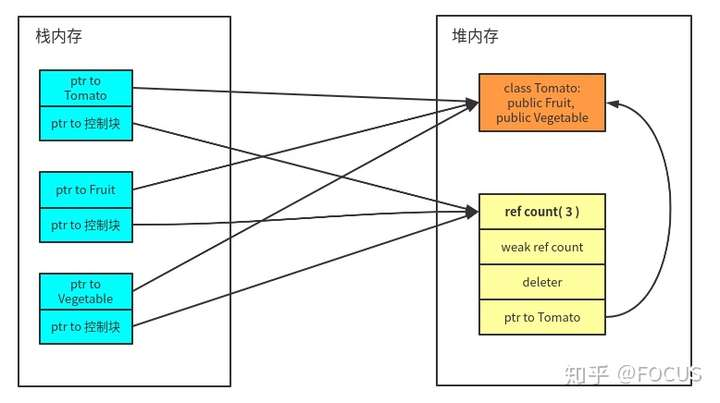template< class Y >shared_ptr( const shared_ptr<Y>& r, element_type* ptr ) noexcept;


Aliasing constructor，简单说就是构造出来的 shared_ptr 对象和参数 r 指向同一个控制块（会影响 r 指向的资源的生命周期），但是指向共享资源的指针是参数 ptr。看下面这个例子。

using Vec = std::vector<int>;std::shared_ptr<int> GetSPtr() {    auto elts = {0, 1, 2, 3, 4};    std::shared_ptr<Vec> pvec = std::make_shared<Vec>(elts);    return std::shared_ptr<int>(pvec, &(*pvec));}std::shared_ptr<int> sptr = GetSPtr();for (int i = -2; i < 3; ++i) {    printf("%d\n", sptr.get()[i]);}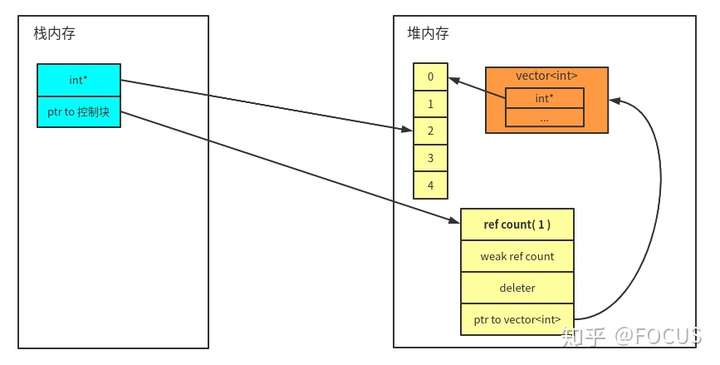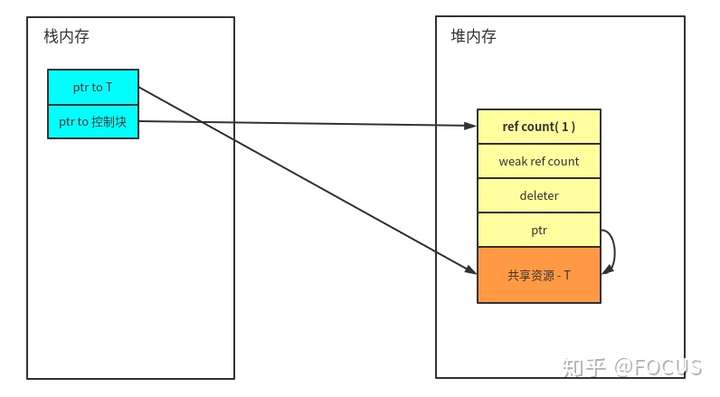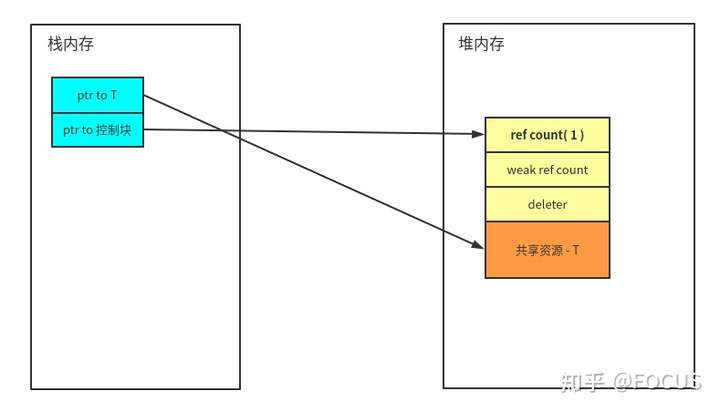## std::weak_ptr

std::weak_ptr 要与 std::shared_ptr 一起使用。 一个 std::weak_ptr 对象看做是 std::shared_ptr 对象管理的资源的观察者，它不影响共享资源的生命周期：

1. 如果需要使用 weak_ptr 正在观察的资源，可以将 weak_ptr 提升为 shared_ptr。
2. 当 shared_ptr 管理的资源被释放时，weak_ptr 会自动变成 nullptr。\
void Observe(std::weak_ptr<int> wptr) {    if (auto sptr = wptr.lock()) {        std::cout << "value: " << *sptr << std::endl;    } else {        std::cout << "wptr lock fail" << std::endl;    }}std::weak_ptr<int> wptr;{    auto sptr = std::make_shared<int>(111);    wptr = sptr;    Observe(wptr);  // sptr 指向的资源没被释放，wptr 可以成功提升为 shared_ptr}Observe(wptr);  // sptr 指向的资源已被释放，wptr 无法提升为 shared_ptr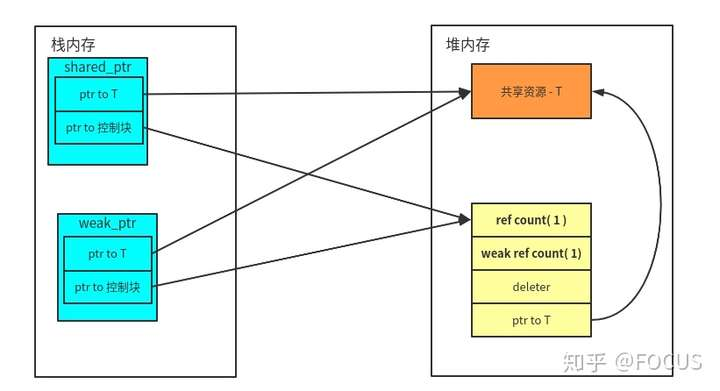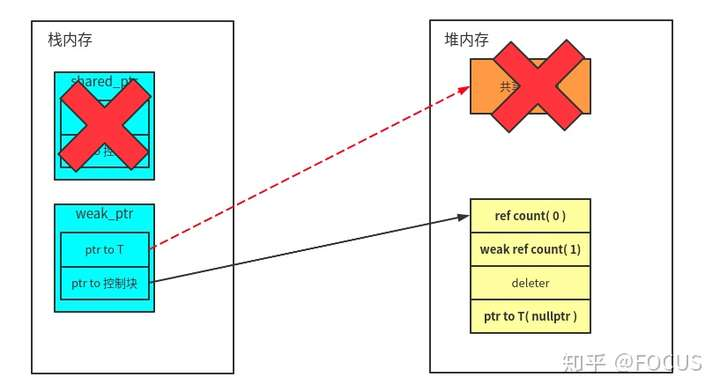## enable_shared_from_this

class Foo { public:  std::shared_ptr<Foo> GetSPtr() {    return std::shared_ptr<Foo>(this);  }};auto sptr1 = std::make_shared<Foo>();assert(sptr1.use_count() == 1);auto sptr2 = sptr1->GetSPtr();assert(sptr1.use_count() == 1);assert(sptr2.use_count() == 1);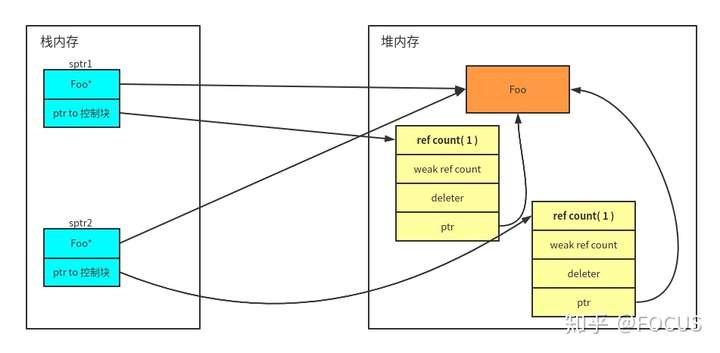class Bar : public std::enable_shared_from_this<Bar> { public:  std::shared_ptr<Bar> GetSPtr() {    return shared_from_this();  }};auto sptr1 = std::make_shared<Bar>();assert(sptr1.use_count() == 1);auto sptr2 = sptr1->GetSPtr();assert(sptr1.use_count() == 2);assert(sptr2.use_count() == 2);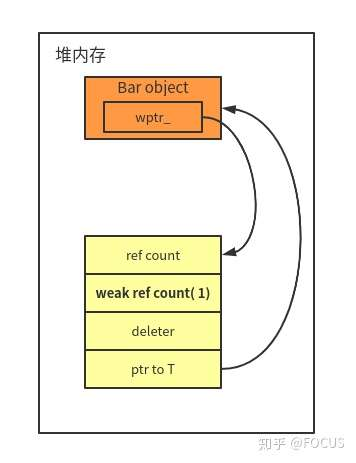auto b = new Bar;auto sptr = b->shared_from_this();


terminate called after throwing an instance of 'std::bad_weak_ptr' what():  bad_weak_ptr


## 小结

1. 当资源是被独占时，使用 std::unique_ptr 对资源进行管理。
2. 当资源会被共享时，使用 std::shared_ptr 对资源进行管理。
3. 使用 std::weak_ptr 作为 std::shared_ptr 管理对象的观察者。
4. 通过继承 std::enable_shared_from_this 来获取 this 的 std::shared_ptr 对象。

## 参考资料kerbal